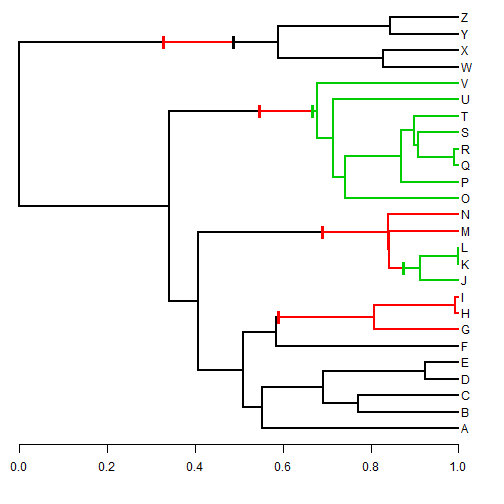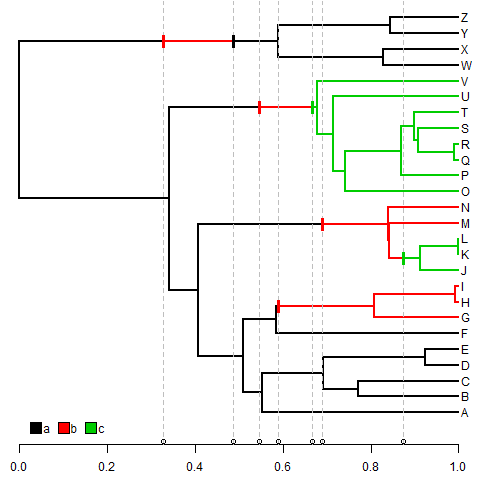## Saturday, August 8, 2015

### Getting the timing of trait changes from a stochastically mapped discrete character history on the tree

Today I received the following question about stochastic character mapped trees in phytools:

I'm working on a data set where I would like to infer the absolute date associated with a transition from one character state to another on a phylogeny (in my case its a habitat transition in a group of fishes).
I am using SIMMAP to map the binary traits onto a subset of trees from a BEAST analysis…. Basically, I would be happy with simply extracting absolute time of each character transition from each SIMMAP tree. For example, on each tree, I just want to be able to extract that transitions happened at 10.1 mya, 12.5 mya, 18.8 mya, etc…. I just cannot figure out a straight-forward way to do this for 100-1000 trees and I was wondering if there was a function in phytools that was designed for just this sort of thing.

Well, there are several functions in phytools, such as `contSimmap` and `describe.simmap` that, in addition to `densityMap`, can be used to summarize the results of stochastic mapping in phytools; however none contain the precise function that is being sought after here. That said, it should not be too hard.

Let's start by seeing how it can be done on a single history. I'll keep it simple, and simulate a tree with three states: `"a"`, `"b"`, and `"c"`:

``````library(phytools)
Q<-matrix(c(-1,1,0,1,-2,1,0,1,-1),3,3)
colnames(Q)<-rownames(Q)<-letters[1:3]
Q
``````
``````##    a  b  c
## a -1  1  0
## b  1 -2  1
## c  0  1 -1
``````
``````tree<-sim.history(pbtree(n=26,tip.label=LETTERS,
scale=1),anc="a",Q)
``````
``````## Done simulation(s).
``````

This is a simulated history - but this would obviously normally be used with a stochastically mapped discrete character history generated, for example, with the phytools function `make.simmap`.

I'm going to plot the tree with it's mapped discrete character history - but I will also use the function `markChanges` that I just posted on my blog here so that we can see the temporal position of the changes a little bit more easily:

``````plotSimmap(tree,mar=c(4.1,0.1,0.1,0.1))
``````
``````## no colors provided. using the following legend:
##        a        b        c
##  "black"    "red" "green3"
``````
``````axis(1)
## so we can see the changes more clearly:
markChanges<-function(tree,colors=NULL,cex=1,lwd=2){
states<-sort(unique(getStates(tree)))
if(is.null(colors)) colors<-setNames(palette()[1:length(states)],
states)
obj<-get("last_plot.phylo",envir=.PlotPhyloEnv)
nc<-sapply(tree\$maps,length)-1
ii<-which(nc>0)
nc<-nc[ii]
h<-vector()
for(i in 1:length(ii)){
for(j in 1:nc[i]){
ss<-names(tree\$maps[[ii[i]]])[j+1]
mm<-tree\$edge[ii[i],1]
dd<-tree\$edge[ii[i],2]
x<-rep(obj\$xx[mm]+cumsum(tree\$maps[[ii[i]]])[j],2)
y<-c(obj\$yy[dd]-0.5*mean(strheight(LETTERS)*cex),
obj\$yy[dd]+0.5*mean(strheight(LETTERS)*cex))
lines(x,y,lwd=lwd,col=colors[ss],lend=2)
h<-c(h,x)
}
}
invisible(h)
}
markChanges(tree,lwd=3)
``````Now we can use some of the same tricks in the function above to get the heights of all the changes in the tree, along with their types:

``````x<-getStates(tree,"tips")
states<-sort(unique(x))
m<-length(states)
ct<-sapply(states,function(x,y) sapply(y,function(y,x)
paste(x,"->",y,sep=""),x=x),y=states)
ct
``````
``````##   a      b      c
## a "a->a" "b->a" "c->a"
## b "a->b" "b->b" "c->b"
## c "a->c" "b->c" "c->c"
``````
``````ii<-matrix(TRUE,m,m)
diag(ii)<-rep(FALSE,m)
changes<-vector(mode="list",length=m*(m-1))
names(changes)<-as.vector(ct[ii])
nc<-sapply(tree\$maps,length)-1
ind<-which(nc>0)
nc<-nc[ind]
H<-nodeHeights(tree)
maps<-tree\$maps[ind]
for(i in 1:length(maps)){
for(j in 1:nc[i]){
sc<-paste(names(maps[[i]])[j:(j+1)],collapse="->")
h<-H[ind[i],1]+cumsum(maps[[i]])[j]
changes[[sc]]<-c(changes[[sc]],as.numeric(h))
}
}
changes<-changes[!sapply(changes,is.null)]
changes<-lapply(changes,sort)
changes
``````
``````## \$`a->b`
##  0.3263951 0.5449173 0.5896102 0.6901126
##
## \$`b->a`
##  0.4872248
##
## \$`b->c`
##  0.6666082 0.8735417
``````

We should be able to see by plotting the changes on the tree that our computed heights correspond with the mapped changes on our plotted tree:

``````plotSimmap(tree,ylim=c(0,Ntip(tree)),mar=c(4.1,0.1,0.1,0.1))
``````
``````## no colors provided. using the following legend:
##        a        b        c
##  "black"    "red" "green3"
``````
``````xx<-markChanges(tree,lwd=3)
axis(1)
obj<-sapply(xx,function(x) lines(rep(x,2),par()\$usr[3:4],
lty="dashed",col="grey"))
obj<-sapply(changes,function(x) points(x,rep(par()\$usr+0.2,
length(x))))
y=0,vertical=FALSE,prompt=FALSE)
markChanges(tree,lwd=3)
``````Of course, we can duplicate this across a sample of trees from a posterior sample; however we need to be cognizant of the fact that we are sure to end up with different numbers of changes of each type in each different tree.

That's it.

1.1.